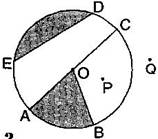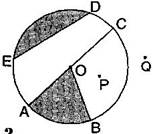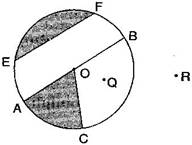1. /
2. CBSE
3. /
4. Class 06
5. /
6. Mathematics
7. /
8. NCERT Solutions for Class...

# NCERT Solutions for Class 6 Maths Exercise 4.5

NCERT solutions for Maths Basic geometrical ideas## NCERT Solutions for Class 6 Maths Basic geometrical ideas

###### Question 1.From the figure, identify:(a)The centre of circle.

(c)A diameter.

(d)A chord.

(e)Two points in the interior.

(f)A point in the exterior.

(g)A sector.

(h)A segment.

(a) O is the centre.

(b) Three radii: OA, OB and OC

(c) A diameter: AC

(d) A chord: ED

(e) Interior points: O, P

(f) Exterior point: Q

(g) A sector: OAB

(h) A segment:NCERT Solutions for Class 6 Maths Exercise 4.5

###### Question 2.(a) Is every diameter of a circle also a chord?

(b) Is every chord of a circle also a diameter?

Answer: (a) Yes, every diameter of a circle is also a chord.

(b) No, every chord of a circle is not a diameter.

###### Question 3.Draw any circle and mark:

(a)Its centre.

(c)A diameter.

(d)A sector.NCERT Solutions for Class 6 Maths Exercise 4.5

###### Question 4.Say true or false:

(a)Two diameters of a circle will necessarily intersect.

(b)The centre of a circle is always in its interior.

(b) True

## NCERT Solutions for Class 6 Maths Exercise 4.5

NCERT Solutions Class 6 Maths PDF (Download) Free from myCBSEguide app and myCBSEguide website. Ncert solution class 6 Maths includes text book solutions from Class 6 Maths Book . NCERT Solutions for CBSE Class 6 Maths have total 14 chapters. 6 Maths NCERT Solutions in PDF for free Download on our website. Ncert Maths class 6 solutions PDF and Maths ncert class 6 PDF solutions with latest modifications and as per the latest CBSE syllabus are only available in myCBSEguide.

## CBSE app for Students

To download NCERT Solutions for Class 6 Maths, Social Science Computer Science, Home Science, Hindi English, Maths Science do check myCBSEguide app or website. myCBSEguide provides sample papers with solution, test papers for chapter-wise practice, NCERT solutions, NCERT Exemplar solutions, quick revision notes for ready reference, CBSE guess papers and CBSE important question papers. Sample Paper all are made available through the best app for CBSE students and myCBSEguide website.Test Generator

Create question paper PDF and online tests with your own name & logo in minutes.myCBSEguide

Question Bank, Mock Tests, Exam Papers, NCERT Solutions, Sample Papers, Notes

### 4 thoughts on “NCERT Solutions for Class 6 Maths Exercise 4.5”

1. It help me a lot

2. Thank you it helps me very much

3. this is notb 4.5 it is 4.6

4. Best website ever know love it ?